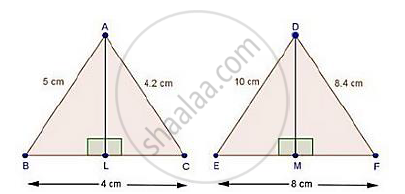Share

# In triangle Abc and triangle Def, It is Being Given That: Ab = 5 Cm, Bc = 4 Cm and Ca = 4.2 Cm; De=10cm, Ef = 8 Cm and Fd = 8.4 Cm. If Al ⊥ Bc and Dm ⊥ Ef, Find Al: Dm. - CBSE Class 10 - Mathematics

ConceptSimilarity Examples and Solutions

#### Question

In ΔABC and ΔDEF, it is being given that: AB = 5 cm, BC = 4 cm and CA = 4.2 cm; DE=10cm, EF = 8 cm and FD = 8.4 cm. If AL ⊥ BC and DM ⊥ EF, find AL: DM.

#### SolutionSince, "AB"/"DE"="BC"/"EF"="AC"/"DE"=1/2

Then, ΔABC ~ ΔDEF                 [By SSS similarity]

Now, In ΔABL ~ ΔDEM

∠B = ∠E                                 [Δ ABC ~ ΔDEF]

∠ALB = ∠DME                       [Each 90°]

Then, ΔABL ~ ΔDEM               [By AA similarity]

therefore"AB"/"DE"="AL"/"DM"                  [Corresponding parts of similar Δ are proportional]

rArr5/10="AL"/"DM"

rArr1/2="AL"/"DM"

Is there an error in this question or solution?

#### Video TutorialsVIEW ALL 

Solution In triangle Abc and triangle Def, It is Being Given That: Ab = 5 Cm, Bc = 4 Cm and Ca = 4.2 Cm; De=10cm, Ef = 8 Cm and Fd = 8.4 Cm. If Al ⊥ Bc and Dm ⊥ Ef, Find Al: Dm. Concept: Similarity Examples and Solutions.
S